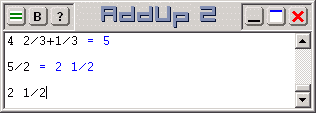# Rational and Fractional Calculations with AddUp 2

Calculations that involve fractions are commonly done with decimal notation, eg. "2.5" to mean two and half. But notation such as "2 1/2" is also supported by AddUp 2 and it is recognized to mean the same thing. There is nothing special to do when entering calculations with this format, as long as it is not ambiguous. For example, you can calculate "2 1/2 * 2" and obtain the result 5, as expected.

The format of the output however is controlled by the current output settings. AddUp offers seven different output formats, including rational and fractional formats. The difference between these is that rational results are given as the ratio of two integer values even if the denominator is greater than the numerator (eg. 3/2) while fractional numbers are given with a whole portion if possible, followed by a fraction where the numerator is smaller than the denominator (eg. 1 1/2). This last format will not show any fraction at all if the numerator is zero.It is important to realize that although results will be shown as an exact fraction, this fraction is simply the best possible approximation of the result given the specified number of decimals used in the fraction. In many cases this fractional result will be exact and correct. But in the case of irrational numbers (such as mathematical constants 'e' or 'pi') then of course the rational value will be an approximation. The value of 'pi' is 3.1415926535897... Using rational output mode this value will be rounded to 22/7 if a single decimal is specified in the format settings. The ratio will be 355/113, a closer approximation, if three decimals are specified instead.